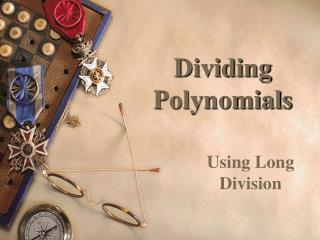# Dividing Polynomials - PowerPoint PPT PresentationDownload PresentationDividing Polynomials

Presentation Description
Download Presentation## Dividing Polynomials

- - - - - - - - - - - - - - - - - - - - - - - - - - - E N D - - - - - - - - - - - - - - - - - - - - - - - - - - -
##### Presentation Transcript

1. Dividing Polynomials Using Long Division

2. Terminology Dividend – Numerator Divisor – Denominator Quotient Remainder

3. Steps to Divide • Divide • Multiply • Subtract • Bring Down • Shampoo, Rinse, Repeat (if necessary)

4. Quick Remembrance 5 1 1 Set up to divide. • Divide 7 by 5. • Multiply 1 by 5. • Subtract down (7–5) • Bring Down 5. • Divide 25 by 5. • Multiply 5 by 5. • Subtract Down (25–25) • Bring Down 5. • Divide 05 by 5. • Multiply 1 by 5. • Subtract Down (5–5) 5 5 25 5 5 Remainder

5. x +1 • Divide x2 by x, which is x. • Multiply x by x + 3, which is x2 + 3x. • Subtract (x2 + 4x) - (x2 + 3x), which is x. • Bring down the 3. • Repeat using x + 3 as our new divisor. 0 Divide x by x, which is 1. Multiply 1 by x + 3, which is x+ 3. Subtract (x + 3) - (x+ 3), which is 0 (remainder).

6. x +2 • Divide x2 by x, which is x. • Multiply x by x + 1, which is x2 + x. • Subtract (x2 + 3x) – (x2 + x), which is 2x. • Bring down the -6. • Repeat using 2x – 6 as our new divisor. -8 Divide x by x, which is 2. Multiply 2 by x + 1, which is 2x+ 2. Subtract (2x – 6) – (2x+ 2), which is -8 (remainder).

7. Divide Multiply Subtract Bring Down Repeat (if necessary)

8. Divide Multiply Subtract Bring Down Repeat (if necessary) Remainder

9. Divide Multiply Subtract Bring Down Repeat (if necessary) Remainder

10. Divide Multiply Subtract Bring Down Repeat (if necessary)

11. Divide Multiply Subtract Bring Down Repeat (if necessary)